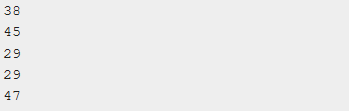## Example for rand function in c programming

rand function in c programming is used to generate the pseudo-random numbers with the help of rand c function.

rand c function return the pseudo-random numbers which is exist between 0 and RAND_MAX.

rand function in c didn't use any parameters.

Syntax for rand c : -

`int rand(void)`

# Example program for rand c

```#include <stdio.h>
#include <stdlib.h>

int main () {
int i, n;
time_t t;

n = 5;

/* Intializes random number generator */
srand((unsigned) time(&t));

/* Print 5 random numbers from 0 to 49 */
for( i = 0 ; i < n ; i++ ) {
printf("%d\n", rand() % 50);
}

return(0);
}```output program for rand c